## Atomic Structure Questions and Answers Part-18

1. The kinetic energy of an electron in the second Bohr orbit of a hydrogen atom is [$a_{0}$ is Bohr radius] :
a) $\frac{h^{2}}{4\pi^{2}ma_0^2}$
b) $\frac{h^{2}}{16\pi^{2}ma_0^2}$
c) $\frac{h^{2}}{32\pi^{2}ma_0^2}$
d) $\frac{h^{2}}{64\pi^{2}ma_0^2}$

Explanation: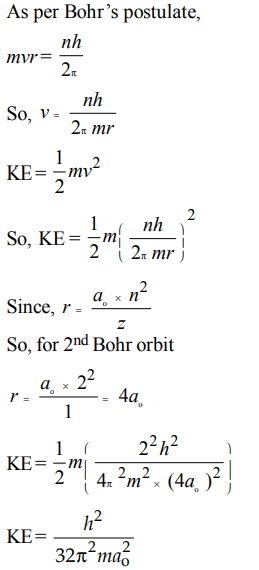2. Given that the abundances of isotopes 54Fe, 56Fe and 57Fe are 5%, 90% and 5%, respectively, the atomic mass of Fe is
a) 55.85
b) 55.95
c) 55.75
d) 56.05

Explanation: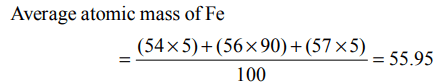3. Which statement is not correct ?
a) Volume of proton is approx $\left(\frac{4}{3}\pi r^{3}\right)1.5*10^{-38}cm^{3}$
b) Radius of $\overline{e}$ is $42.8 ×10^{-13}cm^{3}$
c) Density of nucleus is $10^{14}g/cm^{3}$
d) All are correct

Explanation: All the statements are correct

4. The potential energy of electron present in ground state of $Li^{2+}$ ion is represented by :
a) $\frac{+3e^{2}}{4\pi\epsilon_{0}r}$
b) $\frac{-3e}{4\pi\epsilon_{0}r}$
c) $\frac{-3e^{2}}{4\pi\epsilon_{0}r^{2}}$
d) $\frac{-3e^{2}}{4\pi\epsilon_{0}r}$

Explanation: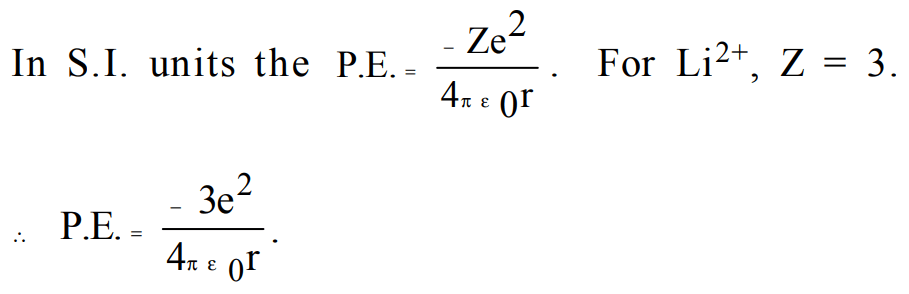5. Which principle/rule limits the maximum no. of electrons in an orbital to two ?
a) Aufbau principle
b) Pauli's exclusion principle
c) Hund's rule of max. multiplicity
d) Heisenberg's uncertainty principle

Explanation: According to Pauli's exclusion principle the maximum number of electrons with opposite spin present in an atomic orbital is two

6. Photoelectric effect is the phenomenon in which
a) photons come out of metal when hit by a beam of electrons
b) photons come out of the nucleus of an atom under the action of an electric field
c) electrons come out of metal with a constant velocity which depends on frequency and intesity of incident light.
d) electrons come out of metal with different velocities not greater than a certain value which depends upon frequency of incident light and not on intensity

Explanation: In photoelectric effect K.E., hence velocity is directly proportional to frequency of the incident light and independent of the intensity of light.

7. If $E_{1},E_{2}andE_{3}$   represent respectively the kinetic energies of an electron and an alpha particle and a proton each having same de-broglie wavelength then
a) $E_{1}>E_{3}>E_{2}$
b) $E_{2}>E_{3}>E_{1}$
c) $E_{1}>E_{2}>E_{3}$
d) $E_{1}=E_{2}=E_{3}$

Explanation: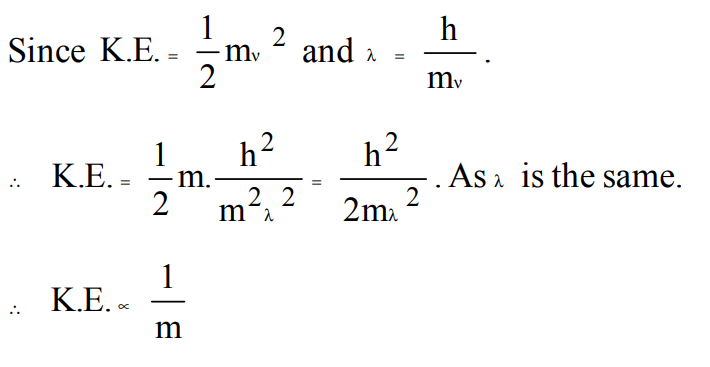8. The angular speed of the electron in nth orbit of Bohr hydrogen atom is
a) directly proportional to n
b) inversely proportional of $\sqrt{n}$
c) inversely proportional to $n^{2}$
d) inversely proportional to $n^{3}$

Explanation: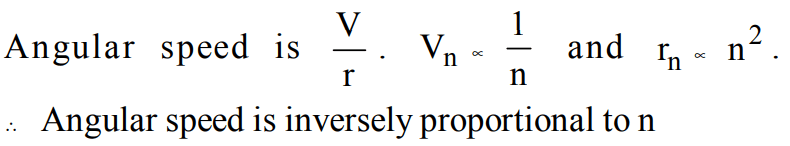9. The Bohr's energy equation for H atom reveals that the energy level of a shell is given by $E = –13.58/n^{2}eV$  . The smallest amount that an H atom will absorb if in ground state is
a) 1.0 eV
b) 3.39 eV
c) 6.79 eV
d) 10.19 eV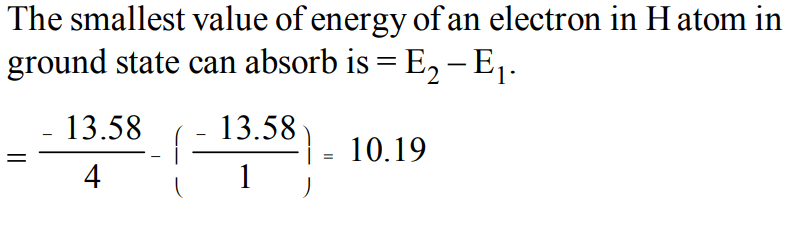10. If the Planck's constant $h = 6.6 ×10^{-34}Js$    , the de-Broglie's wavelength of a particle having momentum of $3.3 ×10^{-24}m/s$     will be